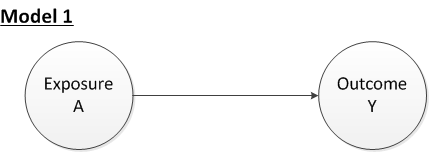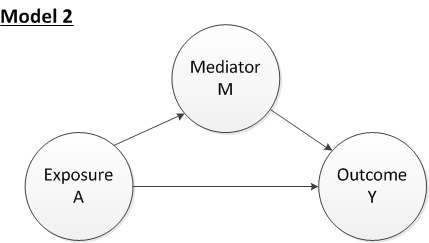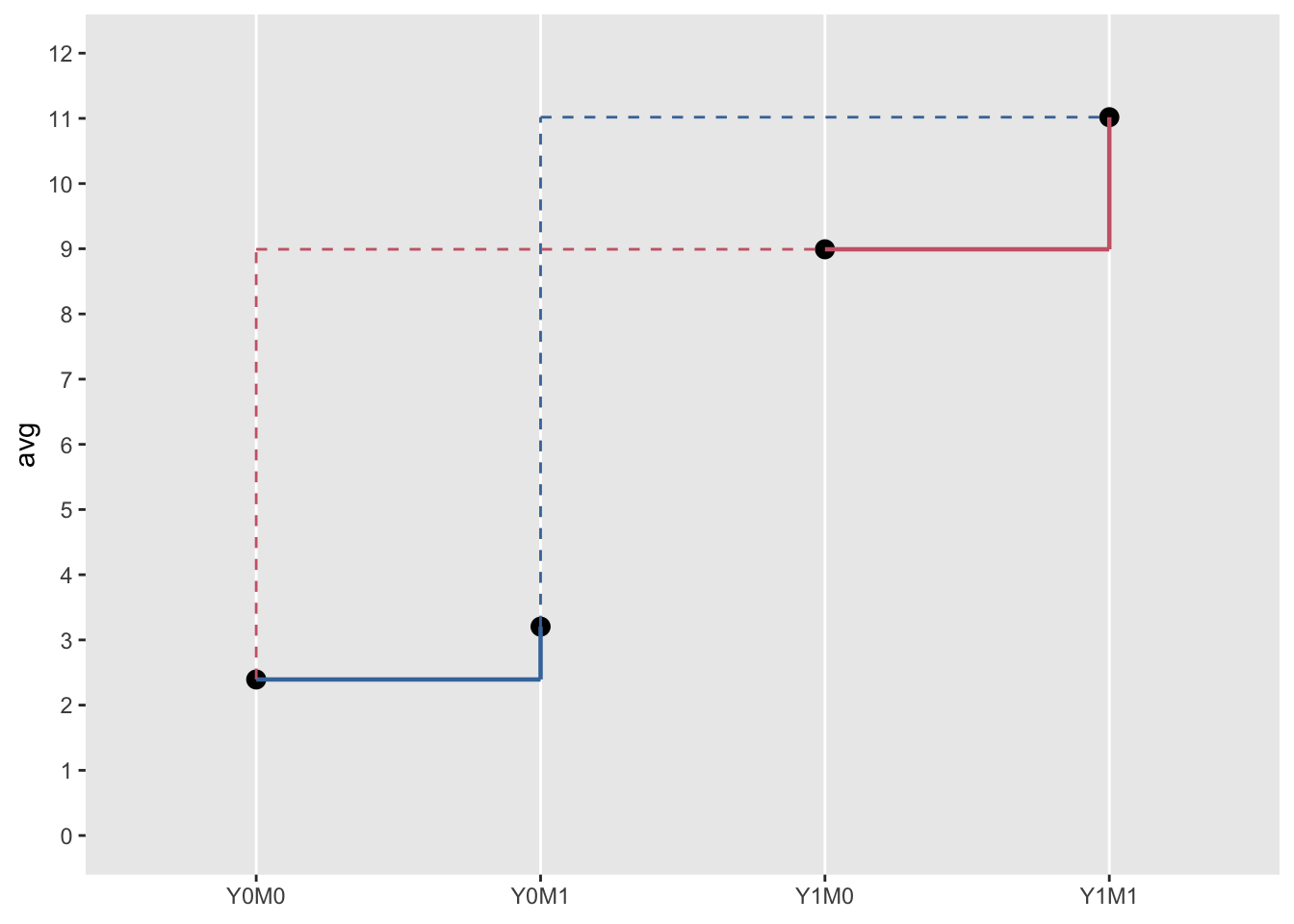# Causal mediation estimation measures the unobservable

I put together a series of demos for a group of epidemiology students who are studying causal mediation analysis. Since mediation analysis is not always so clear or intuitive, I thought, of course, that going through some examples of simulating data for this process could clarify things a bit.

Quite often we are interested in understanding the relationship between an exposure or intervention on an outcome. Does exposure $$A$$ (could be randomized or not) have an effect on outcome $$Y$$?But sometimes we are interested in understanding more than whether or not $$A$$ causes or influences $$B$$; we might want to have some insight into the mechanisms underlying that influence. And this is where mediation analysis can be useful. (If you want to delve deeply into the topic, I recommend you check out this book by Tyler VanderWeele, or this nice website developed at Columbia University.)

In the example here, I am using the simplest possible scenario of an exposure $$A$$, a mediator $$M$$, and an outcome $$Y$$, without any confounding:A key challenge of understanding and conducting a mediation analysis is how we should quantify this concept of mediation. Sure, $$A$$ has an effect on $$M$$, which in turn has an effect on $$Y$$, and $$A$$ also may have an effect on $$Y$$ through other pathways. But how can we make sense of all of this? One approach, which is a relatively recent development, is to use the potential outcome framework of causal inference to define the various estimands (or quantities) that arise in a mediation analysis. (I draw on a paper by Imai, Keele and Yamamoto for the terminology, as there is not complete agreement on what to call various quantities. The estimation methods and software used here are also described in the paper.)

### Defining the potential outcomes

In an earlier post, I described the concept of potential outcomes. I extend that a bit here to define the quantities we are interested in. In this case, we have two effects of the possible exposure: $$M$$ and $$Y$$. Under this framework, each individual has a potential outcome for each level of $$A$$ (I am assuming $$A$$ is binary). So, for the mediator, $$M_{i0}$$ and $$M_{i1}$$ are the values of $$M$$ we would observe for individual $$i$$ without and with exposure, respectively. That is pretty straightforward. (From here on out, I will remove the subscript $$i$$, because it gets a little unwieldy.)

The potential outcomes under $$Y$$ are less intuitive, as there are four of them. First, there is $$Y_{0M_0}$$, which is the potential outcome of $$Y$$ without exposure for $$A$$ and whatever the potential outcome for $$M$$ is without exposure for $$A$$. This is what we observe when $$A=0$$ for an individual. $$Y_{1M_1}$$ is the potential outcome of $$Y$$ with exposure for $$A$$ and whatever the potential outcome for $$M$$ is with exposure for $$A$$. This is what we observe when $$A=1$$ for an individual. That’s all fine.

But we also have $$Y_{0M_1}$$, which can never be observed unless we can intervene on the mediator $$M$$ somehow. This is the potential outcome of $$Y$$ without exposure for $$A$$ and whatever the mediator would have been had the individual been exposed. This potential outcome is controversial, because it is defined across two different universes of exposure to $$A$$. Finally, there is $$Y_{1M_0}$$. It is analogously defined across two universes, but in reverse.

### Defining the causal mediation effects and direct effects

The estimands or quantities that we are interested are defined in terms of the potential outcomes. The causal mediation effects for an individual are

\begin{aligned} CME_0 &= Y_{0M_1} - Y_{0M_0} \\ CME_1 &= Y_{1M_1} - Y_{1M_0}, \end{aligned}

and the causal direct effects are

\begin{aligned} CDE_0 &= Y_{1M_0} - Y_{0M_0} \\ CDE_1 &= Y_{1M_1} - Y_{0M_1}. \end{aligned}

A few important points. (1) Since we are in the world of potential outcomes, we do not observe these quantities for everyone. In fact, we don’t observe these quantities for anyone, since some of the measures are across two universes. (2) The two causal mediation effects under do not need to be the same. The same goes for the two causal direct effects. (3) Under a set of pretty strong assumptions related to unmeasured confounding, independence, and consistency (see Imai et al for the details), the average causal mediation effects and average causal direct effects can be estimated using observed data only. Before I simulate some data to demonstrate all of this, here is the definition for the total causal effect (and its decomposition into mediation and direct effects):

\begin{aligned} TCE &= Y_{1M_1} - Y_{0M_0} \\ &= CME_1 + CDE_0 \\ &= CME_0 + CDE_1 \end{aligned}

### Generating the data

I’m using the simstudy package to generate the data. I’ll start by generating the binary potential outcomes for the mediator, $$M_0$$ and $$M_1$$, which are correlated in this example. $$P(M_1=1) > P(M_0=1)$$, implying that exposure to $$A$$ does indeed have an effect on $$M$$. Note that it is possible that for an individual $$M_0 = 1$$ and $$M_1 = 0$$, so that exposure to $$A$$ has an effect contrary to what we see in the population generally. (We don’t need to make this assumption in the data generation process; we could force $$M_1$$ to be 1 if $$M_0$$ is 1.)

set.seed(3872672)
dd <- genCorGen(n=5000, nvars = 2,  params1 = c(.2, .6),
dist = "binary", rho = .3, corstr = "cs",
wide = TRUE, cnames = c("M0", "M1"))

Observe treatment:

dd <- trtObserve(dd, 0.6, grpName = "A")

Initial data set:

dd
##         id A M0 M1
##    1:    1 0  0  1
##    2:    2 1  0  0
##    3:    3 1  0  1
##    4:    4 0  1  1
##    5:    5 1  0  0
##   ---
## 4996: 4996 1  0  1
## 4997: 4997 0  0  0
## 4998: 4998 1  1  1
## 4999: 4999 1  1  0
## 5000: 5000 0  0  0

$$Y_{0M_0}$$ is a function of $$M_0$$ and some noise $$e_0$$, and $$Y_{0M_1}$$ is a function of $$M_1$$ and the same noise (this is not a requirement). However, if $$M_0 = M_1$$ (i.e. the mediator is not affected by exposure status), then I am setting $$Y_{0M_1} = Y_{0M_0}$$. In this case, $$CME_0$$ for an individual is $$2(M_1 - M_0)$$, so $$CME_0 \in \{-2, 0, 2\}$$, and the population average $$CME_0$$ will depend on the mixture of potential outcomes $$M_0$$ and $$M_1$$.

def <- defDataAdd(varname = "e0", formula = 0,
variance = 1, dist = "normal")
def <- defDataAdd(def, varname = "Y0M0", formula = "2 + M0*2 + e0",
dist = "nonrandom")
def <- defDataAdd(def, varname = "Y0M1", formula = "2 + M1*2 + e0",
variance = 1, dist = "nonrandom")

The same logic holds for $$Y_{1M_0}$$ and $$Y_{1M_1}$$, though at the individual level $$CME_1 \in \{-5, 0, 5\}$$:

def <- defDataAdd(def, varname = "e1", formula = 0,
variance = 1, dist = "normal")
def <- defDataAdd(def, varname = "Y1M0", formula = "8 + M0*5 + e1",
dist = "nonrandom")
def <- defDataAdd(def, varname = "Y1M1", formula = "8 + M1*5 + e1",
dist = "nonrandom")

The observed mediator ($$M$$) and outcome ($$Y$$) are determined by the observed exposure ($$A$$).

def <- defDataAdd(def, varname = "M",
formula = "(A==0) * M0 + (A==1) * M1", dist = "nonrandom")
def <- defDataAdd(def, varname = "Y",
formula = "(A==0) * Y0M0 + (A==1) * Y1M1", dist = "nonrandom")

Here is the entire data definitions table:

e0 0 1 normal identity
Y0M0 2 + M0*2 + e0 0 nonrandom identity
Y0M1 2 + M1*2 + e0 1 nonrandom identity
e1 0 1 normal identity
Y1M0 8 + M0*5 + e1 0 nonrandom identity
Y1M1 8 + M1*5 + e1 0 nonrandom identity
M (A==0) * M0 + (A==1) * M1 0 nonrandom identity
Y (A==0) * Y0M0 + (A==1) * Y1M1 0 nonrandom identity

Based on the parameters used to generate the data, we can calculate the expected causal mediation effects:

\begin{aligned} E[CME_0] &= E[2 + 2M_1 + e_0] - E[2+2M_0+e_0] \\ &= E[2(M_1 - M_0)] \\ &= 2(E[M_1] - E[M_0]) \\ &= 2(0.6 - 0.2) \\ &= 0.8 \end{aligned}

\begin{aligned} E[CME_1] &= E[8 + 5M_1 + e_1] - E[8+5M_0+e_1] \\ &= E[5(M_1 - M_0)] \\ &= 5(E[M_1] - E[M_0]) \\ &= 5(0.6 - 0.2) \\ &= 2.0 \end{aligned}

Likewise, the expected values of the causal direct effects can be calculated:

\begin{aligned} E[CDE_0] &= E[8 + 5M_0 + e_1] - E[2+2M_0+e_0] \\ &= E[6 + 5M_0 - 2M_0)] \\ &= 6 + 3M_0 \\ &= 6 + 3*0.2 \\ &= 6.6 \end{aligned}

\begin{aligned} E[CDE_1] &= E[8 + 5M_1 + e_1] - E[2+2M_1+e_0] \\ &= E[6 + 5M_1 - 2M_1)] \\ &= 6 + 3M_1 \\ &= 6 + 3*0.6 \\ &= 7.8 \end{aligned}

Finally, the expected total causal effect is:

\begin{aligned} ATCE &= E[CDE_0] + E[CME_1] = 6.6 + 2.0 \\ &= E[CDE_1] + E[CME_0] = 7.8 + 0.8 \\ &= 8.6 \end{aligned} And now, the complete data set can be generated.

dd <- addColumns(def, dd)
dd <- delColumns(dd, c("e0", "e1")) # these are not needed
dd
##         id A M0 M1  Y0M0 Y0M1  Y1M0  Y1M1 M      Y
##    1:    1 0  0  1 0.933 2.93  7.58 12.58 0  0.933
##    2:    2 1  0  0 2.314 2.31  6.84  6.84 0  6.841
##    3:    3 1  0  1 3.876 5.88  9.05 14.05 1 14.053
##    4:    4 0  1  1 5.614 5.61 12.04 12.04 1  5.614
##    5:    5 1  0  0 1.469 1.47  8.81  8.81 0  8.809
##   ---
## 4996: 4996 1  0  1 2.093 4.09  8.82 13.82 1 13.818
## 4997: 4997 0  0  0 1.734 1.73  7.28  7.28 0  1.734
## 4998: 4998 1  1  1 3.256 3.26 12.49 12.49 1 12.489
## 4999: 4999 1  1  0 5.149 3.15 12.57  7.57 0  7.572
## 5000: 5000 0  0  0 1.959 1.96  5.23  5.23 0  1.959

### Looking at the “observed” potential outcomes

The advantage of simulating data is that we can see what the average causal effects are based on the potential outcomes. Here are the average potential outcomes in the generated data set:

dd[,.( Y0M0 = mean(Y0M0), Y0M1 = mean(Y0M1),
Y1M0 = mean(Y1M0), Y1M1 = mean(Y1M1))]
##    Y0M0 Y0M1 Y1M0 Y1M1
## 1: 2.39  3.2 8.99   11

The four average causal effects based on the data are quite close to the expected values:

dd[, .(ACME0 = mean(Y0M1 - Y0M0), ACME1= mean(Y1M1 - Y1M0),
ACDE0 = mean(Y1M0 - Y0M0), ACDE1= mean(Y1M1 - Y0M1))]
##    ACME0 ACME1 ACDE0 ACDE1
## 1:  0.81  2.03   6.6  7.81

And the here is the average total causal effect from the data set:

dd[, mean(Y1M1 - Y0M0)]
##  8.62

All of these quantities can be visualized in this figure. The lengths of the solid vertical lines are the mediated effects. The lengths of the dotted vertical lines are the direct effects. And the sums of these vertical lines (by color) each represent the total effect:### Estimated causal mediation effect from observed data

Clearly, the real interest is in estimating the causal effects from data that we can actually observe. And that, of course, is where things start to get challenging. I will not go into the important details here (again, Imai et al provide these), but here are formulas that have been derived to estimate the effects (simplified since there are no confounders in this example) and the calculations using the observed data:

$\small \hat{CME_0} =\sum_{m\in0,1}E[Y|A=0, M=m][P(M=m|A=1)-P(M=m|A=0)]$

# Estimate CME0

dd[M == 0 & A == 0, mean(Y)] *
(dd[A == 1, mean(M == 0)] - dd[A == 0, mean(M == 0)]) +
dd[M == 1 & A == 0, mean(Y)] *
(dd[A == 1, mean(M == 1)] - dd[A == 0, mean(M == 1)])
##  0.805

$\small \hat{CME_1} =\sum_{m\in0,1}E[Y|A=1, M=m][P(M=m|A=1)-P(M=m|A=0)]$

# Estimate CME1

dd[M == 0 & A == 1, mean(Y)] *
(dd[A == 1, mean(M == 0)] - dd[A == 0, mean(M == 0)]) +
dd[M == 1 & A == 1, mean(Y)] *
(dd[A == 1, mean(M == 1)] - dd[A == 0, mean(M == 1)])
##  2

$\small \hat{CDE_0} =\sum_{m\in0,1}(E[Y|A=1, M=m] - E[Y|A=0, M=m])P(M=m|A=0)$

# Estimate CDE0

(dd[M == 0 & A == 1, mean(Y)] -  dd[M == 0 & A == 0, mean(Y)]) *
dd[A == 0, mean(M == 0)] +
(dd[M == 1 & A == 1, mean(Y)] -  dd[M == 1 & A == 0, mean(Y)]) *
dd[A == 0, mean(M == 1)]
##  6.56

$\small \hat{CDE_1} =\sum_{m\in0,1}(E[Y|A=1, M=m] - E[Y|A=0, M=m])P(M=m|A=1)$

# Estimate CDE1

(dd[M == 0 & A == 1, mean(Y)] -  dd[M == 0 & A == 0, mean(Y)]) *
dd[A == 1, mean(M == 0)] +
(dd[M == 1 & A == 1, mean(Y)] -  dd[M == 1 & A == 0, mean(Y)]) *
dd[A == 1, mean(M == 1)]
##  7.76

### Estimation with mediation package

Fortunately, there is software available to provide these estimates (and more importantly measures of uncertainty). In R, one such package is mediation, which is available on CRAN. This package implements the formulas derived in the Imai et al paper.

Not surprisingly, the model estimates are in line with expected values, true underlying effects, and the previous estimates conducted by hand:

library(mediation)

med.fit <- glm(M ~ A, data = dd, family = binomial("logit"))
out.fit <- lm(Y ~ M*A, data = dd)

med.out <- mediate(med.fit, out.fit, treat = "A", mediator = "M",
robustSE = TRUE, sims = 1000)
summary(med.out)
##
## Causal Mediation Analysis
##
## Quasi-Bayesian Confidence Intervals
##
##                          Estimate 95% CI Lower 95% CI Upper p-value
## ACME (control)             0.8039       0.7346         0.88  <2e-16 ***
## ACME (treated)             2.0033       1.8459         2.16  <2e-16 ***
## ADE (control)              6.5569       6.4669         6.65  <2e-16 ***
## ADE (treated)              7.7563       7.6555         7.86  <2e-16 ***
## Total Effect               8.5602       8.4317         8.69  <2e-16 ***
## Prop. Mediated (control)   0.0940       0.0862         0.10  <2e-16 ***
## Prop. Mediated (treated)   0.2341       0.2179         0.25  <2e-16 ***
## ACME (average)             1.4036       1.2917         1.52  <2e-16 ***
## ADE (average)              7.1566       7.0776         7.24  <2e-16 ***
## Prop. Mediated (average)   0.1640       0.1524         0.17  <2e-16 ***
## ---
## Signif. codes:  0 '***' 0.001 '**' 0.01 '*' 0.05 '.' 0.1 ' ' 1
##
## Sample Size Used: 5000
##
##
## Simulations: 1000